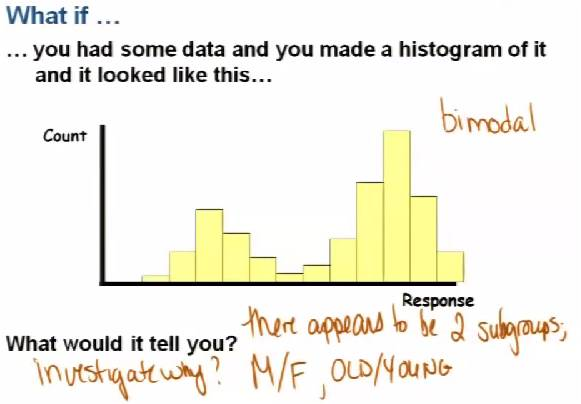# InfoCoBuild

## Statistics 250 - Introduction to Statistics and Data Analysis

Statistics 250: Introduction to Statistics and Data Analysis (Fall 2011, Open.Michigan). Instructor: Brenda Gunderson. This class covers applied statistical methodology from an analysis-of-data viewpoint. Topics covered include frequency distributions; measures of location; mean, median, mode; measures of dispersion; variance; graphic presentation; elementary probability; populations and samples; sampling distributions; one sample univariate inference problems, and two sample problems; categorical data; regression and correlation; and analysis of variance. Use of computers in data analysis is also explored. (from open.umich.edu)Lecture 01 - Turning Data into Information Lecture 02 - Sampling and Gathering Data Lecture 03 - Probability Lecture 04 - Discrete Random Variables Lecture 05 - Continuous Random Variables Lecture 06 - Normal Distribution, Introduction to Sampling Distributions Lecture 07 - Confidence Interval for p Lecture 08 - CI for p and HT for p Lecture 09 - CI and HT for Difference in Two Proportions Lecture 10 - HT for p, Errors, and Power Lecture 11 - Sampling Distribution of Sample Mean Lecture 12 - CI for a Population Mean Lecture 13 - Exam 1 Review Lecture 14 - HT for a Population Mean Lecture 15 - More HT for a Population Mean Lecture 16 - CI and HT for a Population Mean Difference Lecture 17 - Difference in Two Means Lecture 18 - CI for Difference in Two Means (Indep Samples) Lecture 19 - HT for Difference in Two Means (Indep) and NTS Worksheet Lecture 20 - Introduction to ANOVA (Analysis of Variance) Lecture 21 - More ANOVA Lecture 22 - Regression and Exam 2 Review Lecture 23 - More Regression and More Exam 2 Review Lecture 24 - Exam 2 Review Lecture 25 - Inference for Regression Lecture 26 - More Regression and Full Example Lecture 27 - Chi Square Test of GOF and Homogeneity Lecture 28 - Chi Square Test of Independence Lecture 29 - Final Exam Review

 References Statistics 250 - Introduction to Statistics and Data Analysis Instructor: Brenda Gunderson. Course Materials. This class covers applied statistical methodology from an analysis-of-data viewpoint.